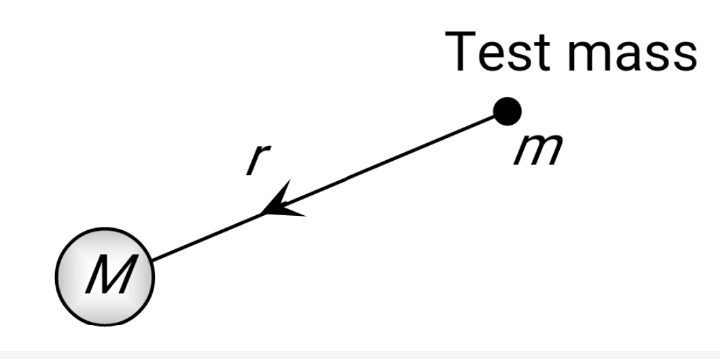# Two bodies of masses are placed at a distance . The gravitational potential at a point on the line joining them where the gravitational field is zero is Option 1) Option 2) Option 3) Option 4)

S Sabhrant Ambastha

As we discussed in

Gravitational field due to Point mass -- wherein

As the distance (r) of test mass from point (M) Increases I decreases.

Option 1)

Incorrect option

Option 2)

Incorrect option

Option 3)

Incorrect option

Option 4)

Exams
Articles
Questions